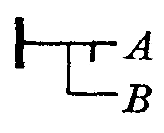## Thue is a matrioshka esoteric computer based on string rewriting rules.

A Thue program consists of two parts: a list of substitution rules, which is terminated with a line having both sides of the operator empty, followed by a string representing the initial program state.

```#::=Unused rules are comments
a::=~Hello Thue!
::=
[a] []
```

Execution consists of picking, from the list of rules, an arbitrary rule whose original string exists as a substring somewhere in the program state, and replacing that substring by the rule's replacement string. This process repeats until there are no rules that can be applied, at which point, the program ends.

```#::=Increment binary number
1_::=1++
0_::=1
01++::=10
11++::=1++0
_0::=_
_1++::=10
::=
_10010011_ _10010100
```

Thue represents one of the simplest possible constraint-based programming language. It is to the constraint-based paradigm what languages like OISC are to the imperative paradigm.### Input

Added to this simple system are two strings which are used to permit Thue to communicate with the outside world. The first of these is the input symbol (":::"). The input symbol is actually the lhs of an implicit rule of which the user (or system's "input stream") is a component. The input symbol, therefore, is replaced by a line of text received from the "input stream."

### Output

As a counterpart of input, the output symbol ("~") is supplied. Like the input symbol, the output symbol triggers an implicit rule which, in this case, encompasses the "output stream." The specific effect is that all text to the right of the output symbol in the rhs of a production is sent to the output stream.

Note that either (or both) of these implicit rules may be overridden by providing explicit rules that perform some other task.

```#::=Sierpinski's triangle, backticks are linebreaks
X::=~_
Y::=~*
Z::=~`
_.::=._X
_*::=*_Y
._|::=.Z-|
*_|::=Z
..-::=.-.
**-::=*-.
*.-::=*-*
.*-::=.-*
@.-::=@_.
@*-::=@_*
::=
@_*...............................|
```
It is pitch black. You are likely to be eaten by a Thue.